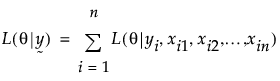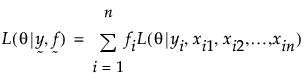Publication date: 07/30/2020

# Frequencies

The impact of frequencies, including those with non-integer values, on an analysis is explained by their effect on the loss function. Suppose that you want to estimate the parameter θ using response values yi and predictors xi1, xi2,..., xin. Suppose that the loss function, assuming no frequency variable, is given by the following:If frequencies fi are defined, then the loss function is:Calculations for all inference-base quantities, such as parameter estimates, standard errors, hypothesis tests, and confidence intervals, are based on this form of the loss function.